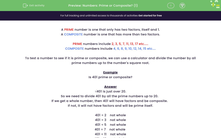# Use Divisibility Rules to Determine Whether or Not a Number is Prime

In this worksheet, students will decide whether a given number is prime or composite.Key stage:  KS 3

Curriculum topic:   Number

Curriculum subtopic:   Use Concepts and Vocabulary for All Numbers

Popular topics:   Numbers worksheets, Prime Numbers worksheets, Factors worksheets

Difficulty level:#### Worksheet Overview

A prime number is one that has only two factors, itself and 1.

A composite number is one that has more than two factors.

Prime numbers include 2, 3, 5, 7, 11, 13, 17 etc.....

Composite numbers include 4, 6, 8, 9, 10, 12, 14, 15 etc....

The number 2 is the only even prime number because all other even numbers will have 2 as a factor.

Sometimes it is easy to spot that a number isn't prime by using divisibility rules:

For example, even numbers end in 0, 2, 4, 6 or 8

Numbers that are divisible by 5 end in a 0 or a 5

Numbers that are divisible by 3 make a multiple of 3 if we add their digits: 243 is divisible by 3 because

2 + 4 + 3 = 9 which is a multiple of 3.

Easy, isn't it?!Sometimes, though, with larger numbers, it can be hard to spot a prime number and that's where the following tip comes in useful!

To test a number to see if it is prime or composite, we can use a calculator and divide the number by all prime numbers up to the number's square root.

Example

Is 401 prime or composite?

√401 is just over 20.

So we need to divide 401 by all the prime numbers up to 20.

If we get a whole number, then 401 will have factors and be composite.

If not, it will not have factors and will be prime itself.

401 ÷ 2    not whole

401 ÷ 3    not whole

401 ÷ 5    not whole

401 ÷ 7    not whole

401 ÷ 11    not whole

401 ÷ 13    not whole

401 ÷ 17    not whole

401 ÷ 19    not whole

401 is prime.

Let's test this out in some questions.

### What is EdPlace?

We're your National Curriculum aligned online education content provider helping each child succeed in English, maths and science from year 1 to GCSE. With an EdPlace account you’ll be able to track and measure progress, helping each child achieve their best. We build confidence and attainment by personalising each child’s learning at a level that suits them.

Get started